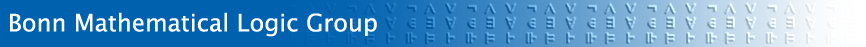## News

Logic and Foundations

Mathematical knowledge is obtained by Proofs, consisting of generally valid and certain conclusions. Mathematical statements are not verified by experiments or social agreements, but they are logically deduced from basic assumptions. This method guarantees the universal applicability of mathematical results: whenever a mathematical statement can be mapped into the physical reality then it holds true.

Euclid's geometry is still the paradigm of a mathematical theory: after the introduction of axioms, postulates, and definitions the geometrical theorems are proved systematically by logical deductions. Pythagoras' theorem is true in the Euclidean system, independently from the question whether the parallel postulate holds in the physical space. The Euclidean method is characterised by its austere, nearly formal language and by the small number of recurring proof principles.

Mathematical logic teaches the formal, axiomatic method and researches its scope and bounds. The formula language of mathematics is in principle absolutely precise, describing computational terms, their relations, and logical dependencies between relations. Intuitive notion of number and size, spatial position, discreteness and continuity, relational and functional dependency have been translated into the mathematical language.

In principle an extremely simple language suffices for all mathematical purposes. Start from equalities and relations between terms. Given two formulas A and B, further formulas can be built using Boolean combinations:

A and B (in short: AB), A or B (AB), A implies B (AB), not AA).

Moreover, free variables of formulas may be quantified:

A holds for all x (∀x A), there exists x such that A (∃x A).

Mathematical reasoning produces correct formulas from axioms which themselves are formulas. Analysing proofs shows that the single steps of a proof are often of a very simple kind, or that they may be cut up into simple steps. As an example, consider the classical modus ponens: If A and if A implies B then B. In symbols:

 A,A→B B
.

This deduction rule possesses a purely formal character: given two appropriate formulas, the formula B is obtained by canceling out the subformula A. Another example is the rule of case distinction

 A→B,¬A→B B
.

This rule is correct, meaning that every Struktur that satisfies the hypotheses AB and ¬AB of the rule also satisfies the conclusion B.

A small number of such deduction rules suffices to form a complete calculus K of mathematical reasoning: if every structur which satisfies the axiom system T also satisfies the formula A gilt, then A can be derived from the formulas of T with the rules of the calculus K. This result is the Gödel completeness theorem which can be viewed as the fundamental theorem of mathematical logic. It is remarkable that the Gödel completeness theorem is itself a mathematical theorem which connects syntactical, nearly algebraic operations on symbol sequences with the class of all mathematical structures. Mathematical logic is the logic of mathematics as well as the mathematics of logic.

Besides the explicitely given axioms, mathematical theories rely on general properties of numbers, of finite and infinite sets, and of functions and relations. This background theory can most easily be formalised as set theory. The Zermelo-Fraenkel axioms of set theory are generally accepted and used as an adequate foundational theory. The question “What is mathematics” can thus be answered in a minimal but comprehensive way: it is the collection of all formulas which can be deduced from the Zermelo-Fraenkel axioms in the calculus K.

Mathematical logic is divided into subareas: Proof theory studies formal languages and deduction calculi. Set theory examines the Zermelo-Fraenkelsche axiom system and its extensions. Model theory considers the class of models of axiom systems. Recursion theory, or computability theory, study the abstract computability of functions.

The Mathematical Logic Group at the university of Bonn specialises in set theory. Set theory is the mathematical theory of the infinite. The strength and universaliy of the axioms allow to formalize formal languages and proofs within the theory. This allows to imitate the liar paradox “this sentence is false” and leads to a statement which cannot be decided inside set theory (Gödel incompleteness theorem).

Further research has shown that some simple properties of infinite sets like “how many real numbers are there” are not decided by the Zermelo-Fraenkel axioms. The undecidability of a statement A can be demonstrated, just like in the foundations of geometry, by exhibiting different models of the axioms, in which A holds in one model and ¬A in another. Such models of set theory can be defined from a given model by restriction or extension.

Research in axiomatic set theory uncovers a multitude of possible mathematical worlds, which differ with respect to their infinitary combinatorics, their properties of real numbers, or even in their theory of natural numbers. Most of these undecidibilites though concern border areas which do not influence everyday mathematical work.

Research at Bonn focusses on models of set theory which are generated by iterations of simple set theoretic operations and on models with large cardinals. We also work in descriptive set theory, studying the set of real numbers. There are close connections between these areas.

Since mathematical logic studies formal languages and arguments it possesses many natural links to computer science, philosophy, and linguistics. Programming languages are formal languages with a semantics which captures computational processes. Databases can be viewed as mathematical structures which can be queried in formal languages. The connection to philosophy is given by the theoretical study of valid argument and by the analysis of the limits of that method. Theoretical linguistics uses formal languages to model natural language. The need for formally and practically trained logicians will grow due to the broad use of formal systems in the modern world.

The Mathematical Logic Group encourages a comprehensive logic education which besides mathematics includes also elements from theoretical computer science, philosophical logic, or formal linguistics.

Last changed: November 18, 2007